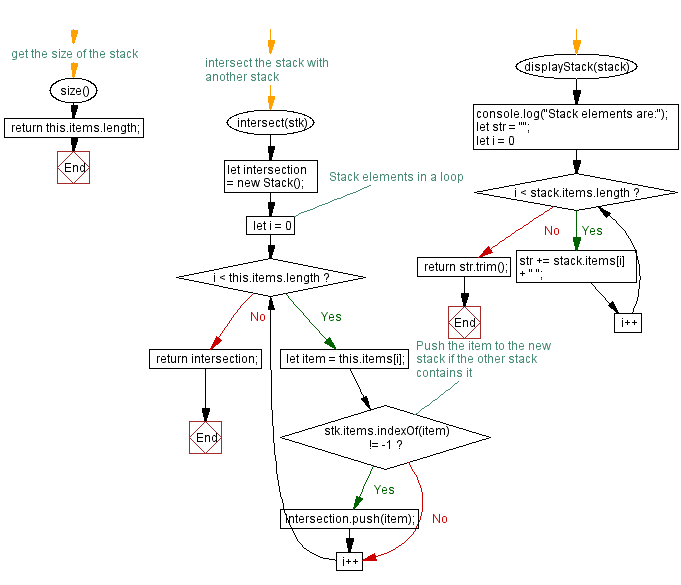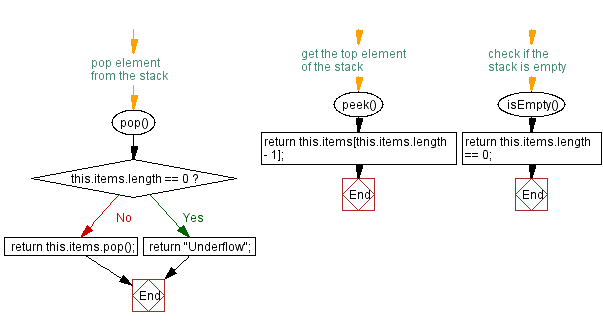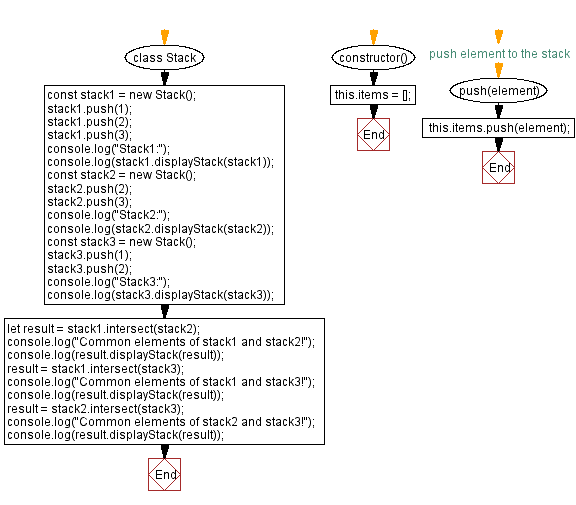# JavaScript Exercises: Find elements that are common in two stacks

## JavaScript Stack: Exercise-28 with Solution

Write a JavaScript program that implements a stack and finds elements that are common in two stacks..

Sample Solution:

JavaScript Code:

``````class Stack {
constructor() {
this.items = [];
}

// push element to the stack
push(element) {
this.items.push(element);
}

// pop element from the stack
pop() {
if (this.items.length == 0) {
return "Underflow";
}
return this.items.pop();
}

// get the top element of the stack
peek() {
return this.items[this.items.length - 1];
}

// check if the stack is empty
isEmpty() {
return this.items.length == 0;
}

// get the size of the stack
size() {
return this.items.length;
}

// intersect the stack with another stack
intersect(stk) {
let intersection = new Stack();

// Stack elements in a loop
for (let i = 0; i < this.items.length; i++) {
let item = this.items[i];

// Push the item to the new stack if the other stack contains it
if (stk.items.indexOf(item) != -1) {
intersection.push(item);
}
}
return intersection;
}
displayStack(stack) {
console.log("Stack elements are:");
let str = "";
for (let i = 0; i < stack.items.length; i++)
str += stack.items[i] + " ";
return str.trim();
}
}
const stack1 = new Stack();
stack1.push(1);
stack1.push(2);
stack1.push(3);
console.log("Stack1:");
console.log(stack1.displayStack(stack1));
const stack2 = new Stack();
stack2.push(2);
stack2.push(3);
console.log("Stack2:");
console.log(stack2.displayStack(stack2));
const stack3 = new Stack();
stack3.push(1);
stack3.push(2);
console.log("Stack3:");
console.log(stack3.displayStack(stack3));
let result = stack1.intersect(stack2);
console.log("Common elements of stack1 and stack2!");
console.log(result.displayStack(result));
result = stack1.intersect(stack3);
console.log("Common elements of stack1 and stack3!");
console.log(result.displayStack(result));
result = stack2.intersect(stack3);
console.log("Common elements of stack2 and stack3!");
console.log(result.displayStack(result));
```
```

Sample Output:

``` "Stack1:"
"Stack elements are:"
"1 2 3"
"Stack2:"
"Stack elements are:"
"2 3"
"Stack3:"
"Stack elements are:"
"1 2"
"Common elements of stack1 and stack2!"
"Stack elements are:"
"2 3"
"Common elements of stack1 and stack3!"
"Stack elements are:"
"1 2"
"Common elements of stack2 and stack3!"
"Stack elements are:"
"2"
```

Flowchart:Live Demo:

See the Pen javascript-stack-exercise-28 by w3resource (@w3resource) on CodePen.

Improve this sample solution and post your code through Disqus

Stack Previous: Checks if two stacks are equal.
Stack Exercises Next: Stack elements in the first but not in the second.

What is the difficulty level of this exercise?

Test your Programming skills with w3resource's quiz.

﻿